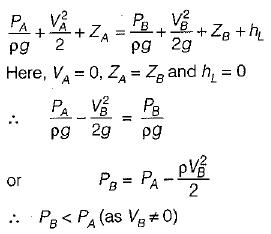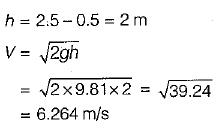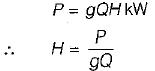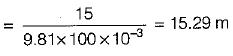Courses

# Test: Dynamic of Fluid Flow - 2

## 10 Questions MCQ Test Topicwise Question Bank for Mechanical Engineering | Test: Dynamic of Fluid Flow - 2

Description
This mock test of Test: Dynamic of Fluid Flow - 2 for Mechanical Engineering helps you for every Mechanical Engineering entrance exam. This contains 10 Multiple Choice Questions for Mechanical Engineering Test: Dynamic of Fluid Flow - 2 (mcq) to study with solutions a complete question bank. The solved questions answers in this Test: Dynamic of Fluid Flow - 2 quiz give you a good mix of easy questions and tough questions. Mechanical Engineering students definitely take this Test: Dynamic of Fluid Flow - 2 exercise for a better result in the exam. You can find other Test: Dynamic of Fluid Flow - 2 extra questions, long questions & short questions for Mechanical Engineering on EduRev as well by searching above.
QUESTION: 1

Solution:
QUESTION: 2

Solution:
QUESTION: 3

### An orifice is known as large orifice when the head of liquid from the center of orifice is

Solution:
QUESTION: 4

In the siphon in figure below assuming ideal flow, the pressure PBSolution:

Applying Bernoulli’s equation between A and BQUESTION: 5

A siphon used to empty a tank consists essentially of a pipe with its summit 0.5 m above the water surface of the tank and its outlet at 2.5 m below the summit. Neglecting friction and other losses, the velocity in the siphon is

Solution:QUESTION: 6

A pump delivers 100 L/s of water and delivers 15 kW of power to the system. The head developed by the pump is

Solution:QUESTION: 7

In a hydropower project a turbine has a head of 50 m. The discharge in the feeding penstock is 3 m3/s. If a head loss of 5 m takes place due to losses, and a power of 1000 kW is extracted, the residual head downstream of the turbine is

Solution:
QUESTION: 8

Venturimeter is used to measure flow of fluids in pipes when pipe is

Solution:
QUESTION: 9

The length of divergent part of venturimeter in comparision to convergent portion is

Solution:
QUESTION: 10

All the terms of energy of Bernoulli’s equation have dimension of

Solution: